Minima/ maxima (statement questions) - Geometry questions

Chapter 6 Class 12 Application of Derivatives
Concept wise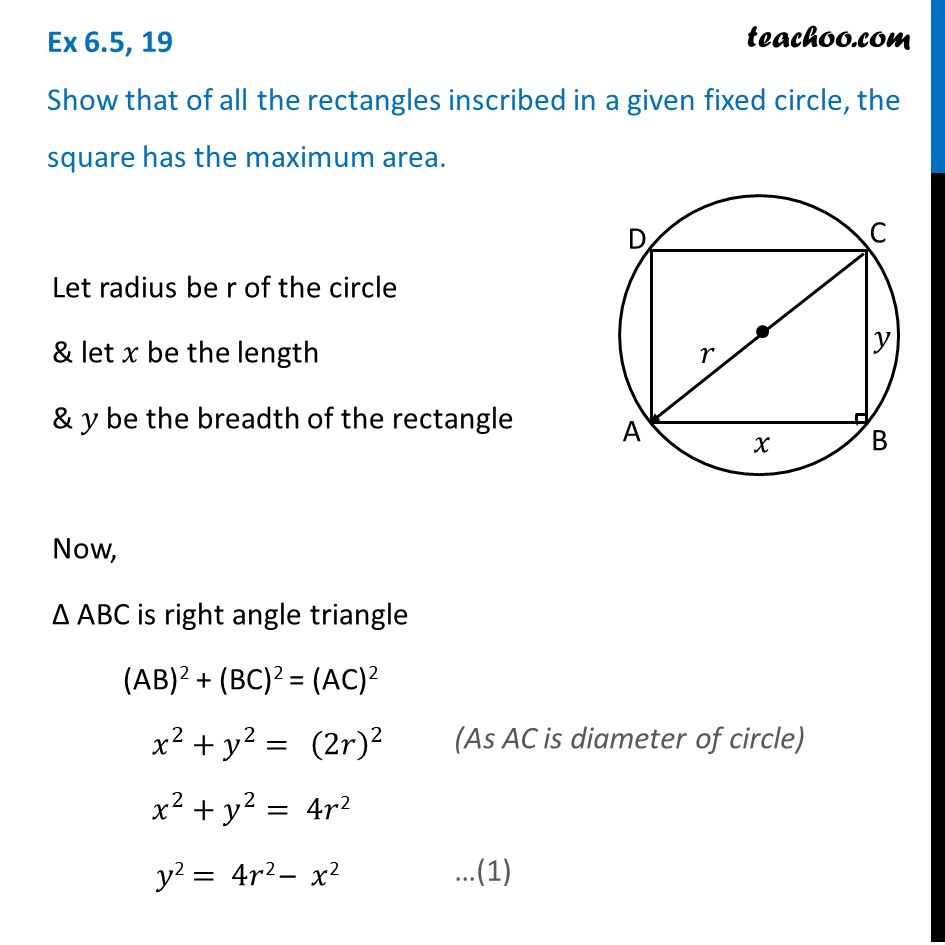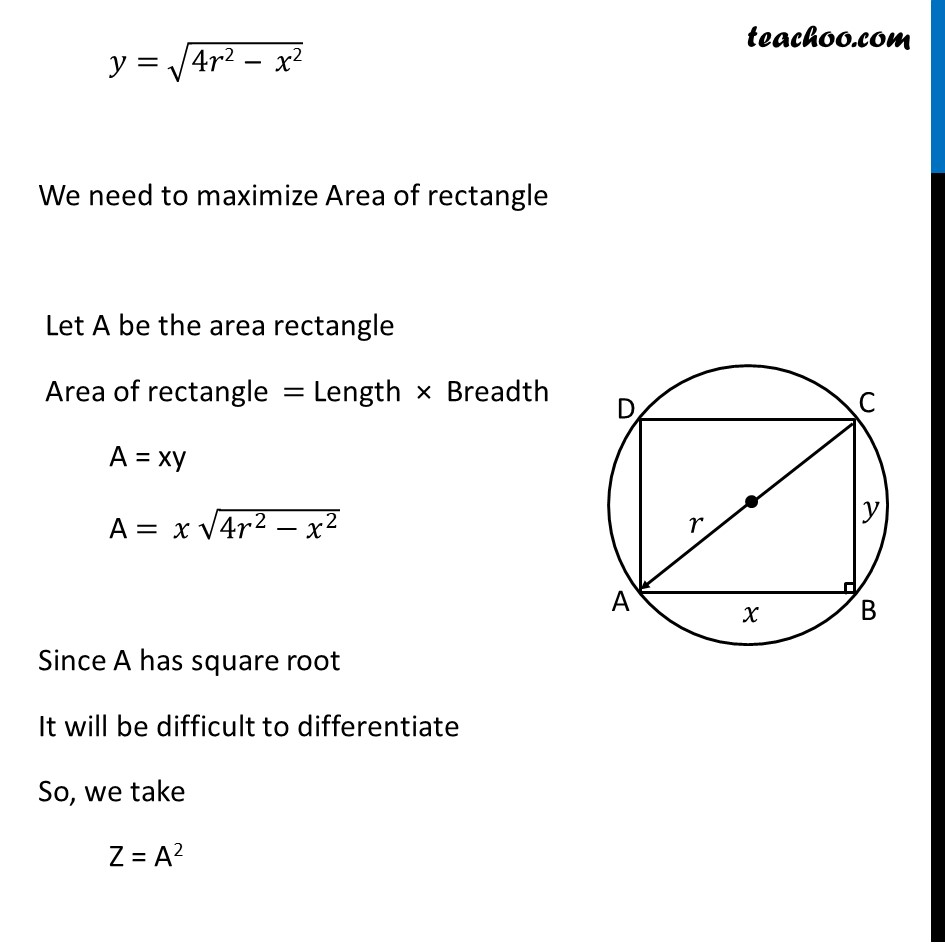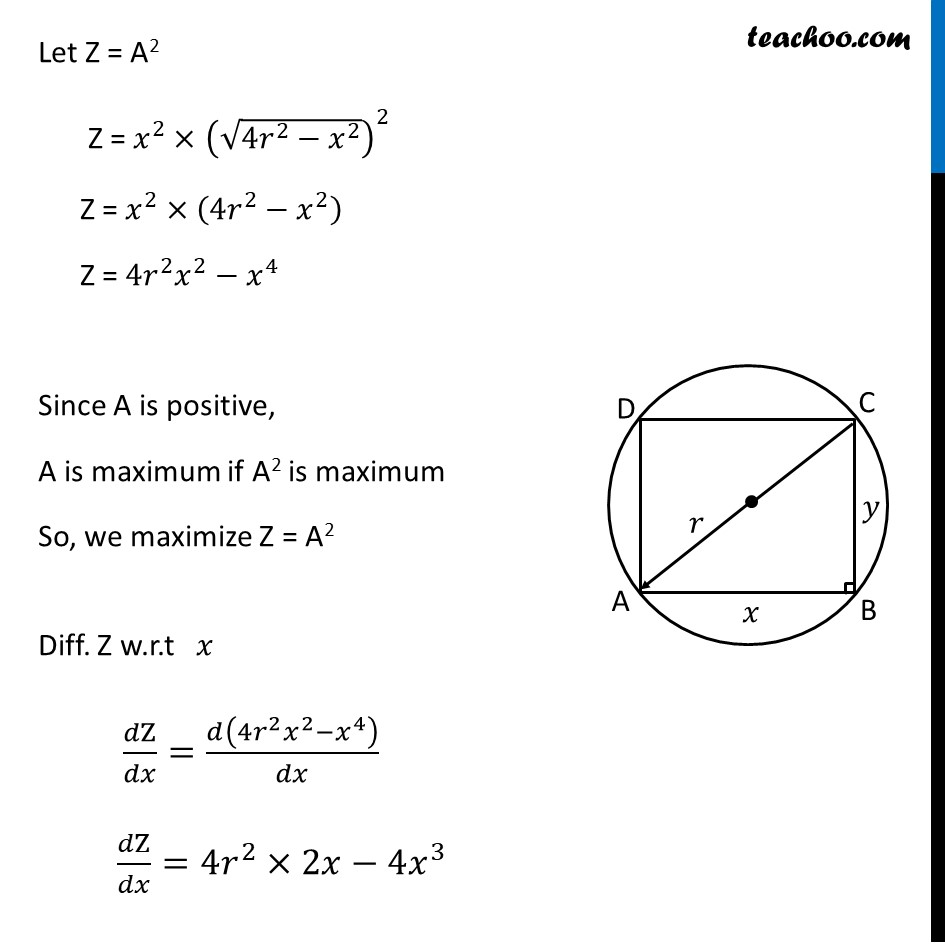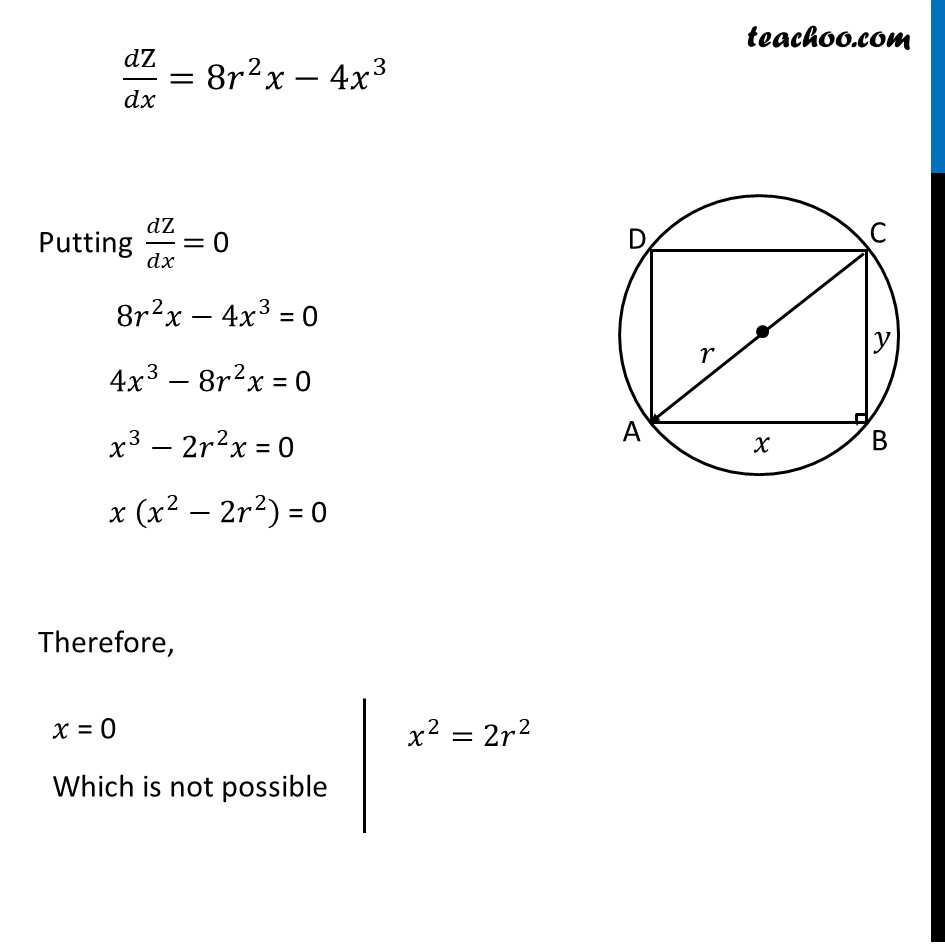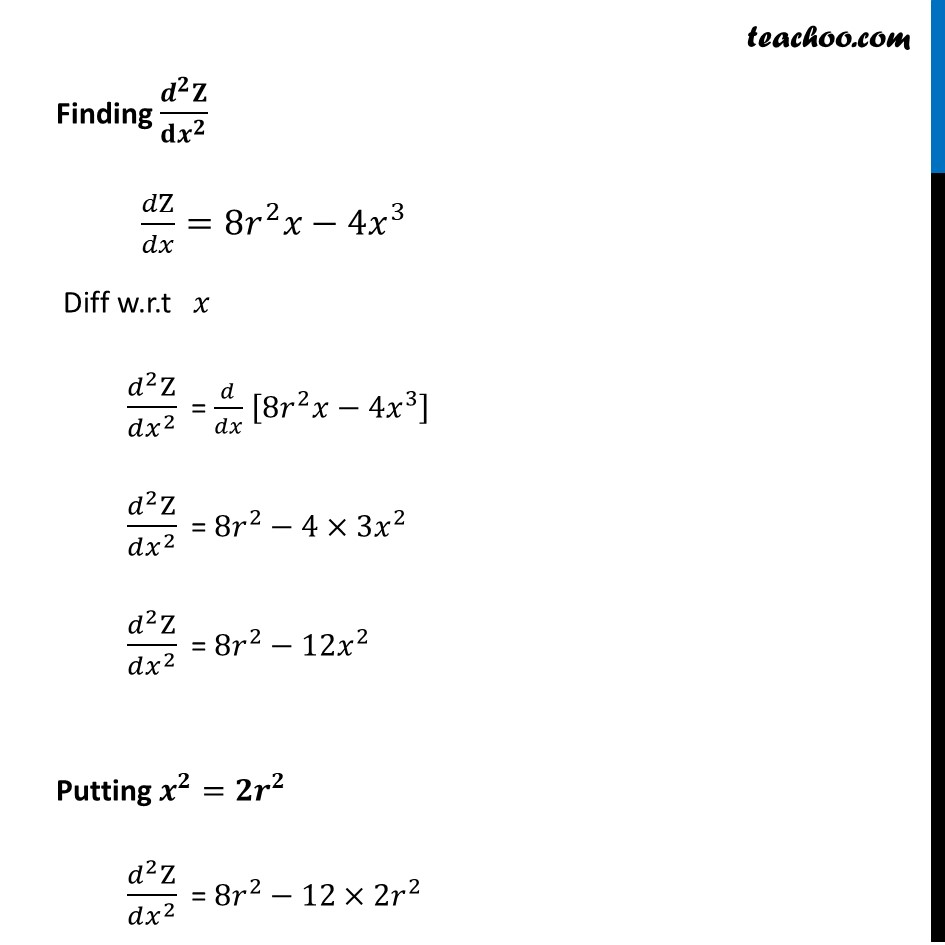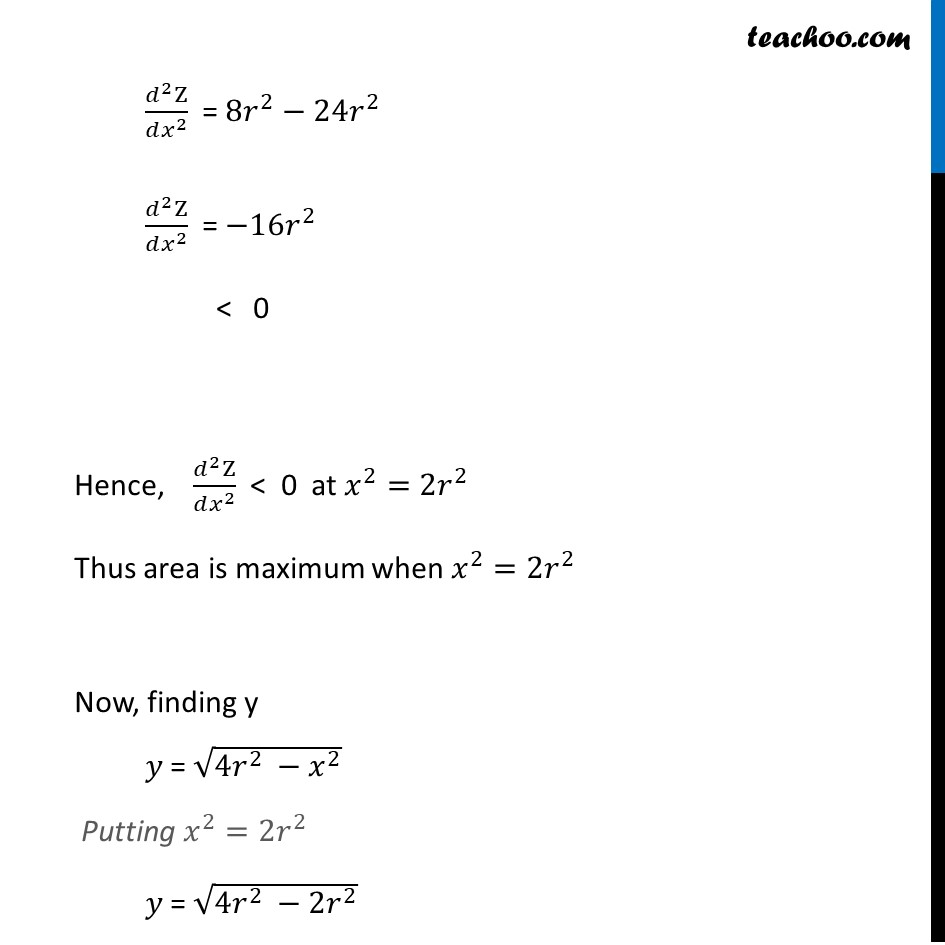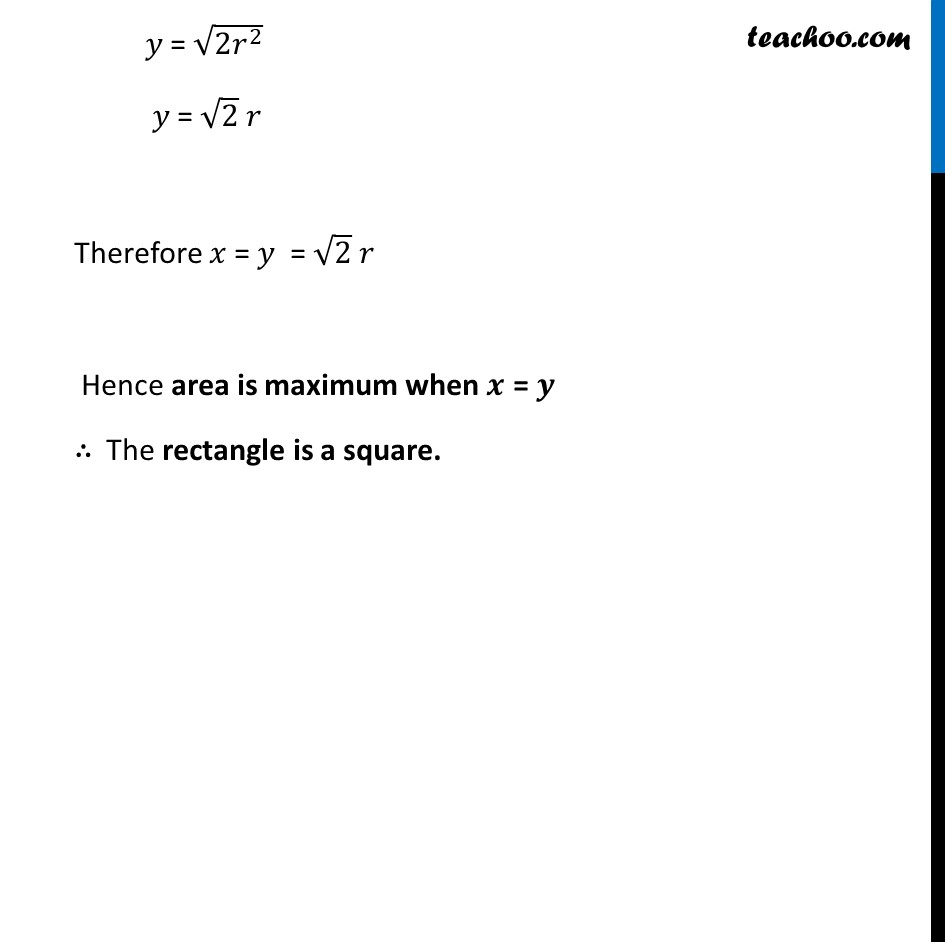Introducing your new favourite teacher - Teachoo Black, at only ₹83 per month

### Transcript

Ex 6.5, 19 Show that of all the rectangles inscribed in a given fixed circle, the square has the maximum area.Let radius be r of the circle & let 𝑥 be the length & 𝑦 be the breadth of the rectangle Now, Δ ABC is right angle triangle (AB)2 + (BC)2 = (AC)2 𝑥^2+𝑦^2 = (2𝑟)^2 𝑥^2+𝑦^2= 4𝑟2 𝑦2 = 4𝑟2 – 𝑥2 (As AC is diameter of circle) 𝑦= √(4𝑟"2 – " 𝑥"2" ) We need to maximize Area of rectangle Let A be the area rectangle Area of rectangle = Length × Breadth A = xy A = 𝑥 √(4𝑟^2−𝑥^2 ) Since A has square root It will be difficult to differentiate So, we take Z = A2 Let Z = A2 Z = 𝑥^2× (√(4𝑟^2−𝑥^2 ))^2 Z = 𝑥^2×(4𝑟^2−𝑥^2 ) Z = 4𝑟^2 𝑥^2−𝑥^4 Since A is positive, A is maximum if A2 is maximum So, we maximize Z = A2 Diff. Z w.r.t 𝑥 𝑑Z/𝑑𝑥=𝑑(4𝑟^2 𝑥^2−𝑥^4 )/𝑑𝑥 𝑑Z/𝑑𝑥=4𝑟^2×2𝑥−4𝑥^3 𝑑Z/𝑑𝑥=8𝑟^2 𝑥−4𝑥^3 Putting 𝑑Z/𝑑𝑥 = 0 8𝑟^2 𝑥−4𝑥^3 = 0 4𝑥^3−8𝑟^2 𝑥 = 0 𝑥^3−2𝑟^2 𝑥 = 0 𝑥 (𝑥^2−2𝑟^2 ) = 0 Therefore, 𝑥 = 0 Which is not possible Finding (𝒅^𝟐 𝐙)/(𝐝𝒙^𝟐 ) 𝑑Z/𝑑𝑥=8𝑟^2 𝑥−4𝑥^3 Diff w.r.t 𝑥 (𝑑^2 Z)/(𝑑𝑥^2 ) = 𝑑/𝑑𝑥 [8𝑟^2 𝑥−4𝑥^3 ] (𝑑^2 Z)/(𝑑𝑥^2 ) = 8𝑟^2−4×3𝑥^2 (𝑑^2 Z)/(𝑑𝑥^2 ) = 8𝑟^2−12𝑥^2 Putting 𝒙^𝟐=𝟐𝒓^𝟐 (𝑑^2 Z)/(𝑑𝑥^2 ) = 8𝑟^2−12×2𝑟^2 (𝑑^2 Z)/(𝑑𝑥^2 ) = 8𝑟^2−24𝑟^2 (𝑑^2 Z)/(𝑑𝑥^2 ) = −16𝑟^2 < 0 Hence, (𝑑^2 Z)/(𝑑𝑥^2 ) < 0 at 𝑥^2=2𝑟^2 Thus area is maximum when 𝑥^2=2𝑟^2 Now, finding y 𝑦 = √(4𝑟^2 −𝑥^2 ) Putting 𝑥^2=2𝑟^2 𝑦 = √(4𝑟^2 −〖2𝑟〗^2 ) 𝑦 = √(〖2𝑟〗^2 ) 𝑦 = √2 𝑟 Therefore 𝑥 = 𝑦 = √2 𝑟 Hence area is maximum when 𝒙 = 𝒚 ∴ The rectangle is a square.